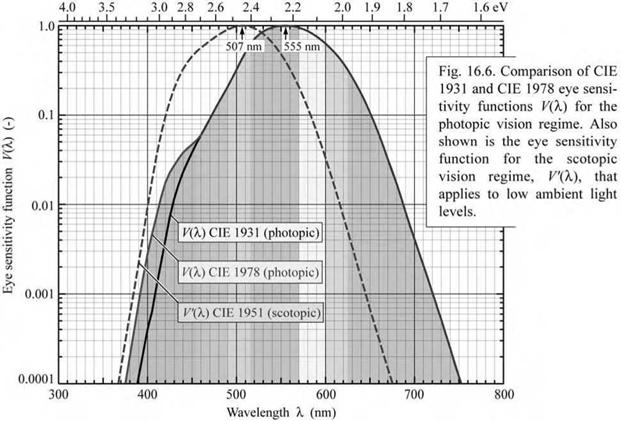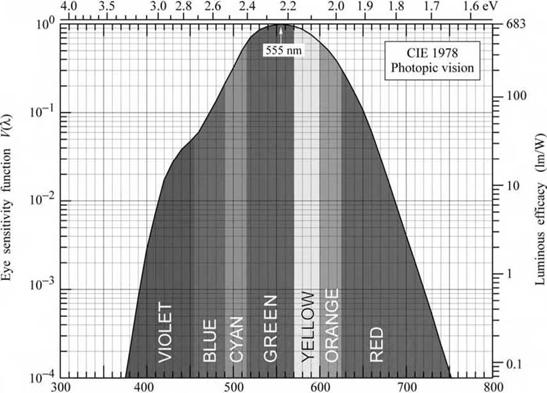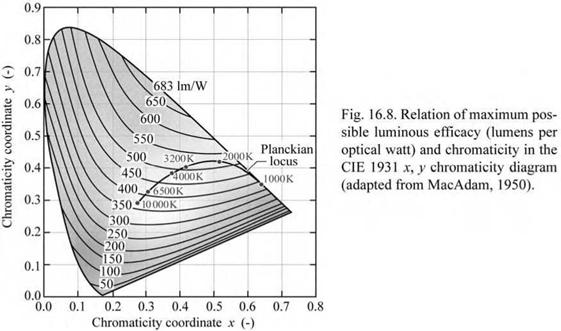## Eye sensitivity function

The conversion between radiometric and photometric units is provided by the luminous efficiency function or eye sensitivity function, V(k). In 1924, the CIE introduced the photopic eye sensitivity function V(k) for point-like light sources where the viewer angle is 2° (CIE, 1931). This function is referred to as the CIE 1931 V(X) function. It is the current photometric standard in the United States.

A modified V(X) function was introduced by Judd and Vos in 1978 (Vos, 1978; Wyszecki and Stiles, 1982, 2000) and this modified function is here referred to as the CIE 1978 V(X) function. The modification was motivated by the underestimation of the human eye sensitivity in the blue and violet spectral region by the CIE 1931 V(k) function. The modified function V(X) has higher values in the spectral region below 460 nm. The CIE has endorsed the CIE 1978 V(k)

function by stating “the spectral luminous efficiency function for a point source may be adequately represented by the Judd modified V(X) function” (CIE, 1988) and “the Judd modified V(X) function would be the preferred function in those conditions where luminance measurements of short wavelengths consistent with color normal observers is desired” (CIE, 1990).The CIE 1931 V(X) function and the CIE 1978 V(X) function are shown in Fig. 16.6. The photopic eye sensitivity function has maximum sensitivity in the green spectral range at 555 nm, where V(X) has a value of unity, i. e. V(555 nm) = 1. Inspection of the figure also reveals that the CIE 1931 V(X) function underestimated the eye sensitivity in the blue spectral range (X < 460 nm). Numerical values of the CIE 1931 and CIE 1978 V(X) function are tabulated in Appendix 16.1.

Also shown in Fig. 16.6 is the scotopic eye sensitivity function V '(X). The peak sensitivity in the scotopic vision regime occurs at 507 nm. This value is markedly shorter than the peak sensitivity in the photopic vision regime. Numerical values of the CIE 1951 V '(X) function are tabulated in Appendix 16.2.

Note that even though the CIE 1978 V(X) function is preferable, it is not the standard, mostly for practical reasons such as possible ambiguities created by changing standards. Wyszecki and Stiles (2000) note that even though the CIE 1978 V(X) function is not a standard, it has been used in several visual studies. The CIE 1978 V(X) function, which can be considered the most accurate description of the eye sensitivity in the photopic vision regime, is shown in Fig. 16.7.Wavelength X (nm) Fig. 16.7. Eye sensitivity function, K(X), (left-hand ordinate) and luminous efficacy, measured in lumens per watt of optical power (right-hand ordinate). V(X) is maximum at 555 nm (after 1978 CIE data).

The eye sensitivity function has been determined by the minimum flicker method, which is the classic method for luminance comparison and for the determination of V(X). The stimulus is a light-emitting small circular area, alternatingly illuminated (with a frequency of 15 Hz) with the standard color and the comparison color. Since the hue-fusion frequency is lower than 15 Hz, the hues fuse. However, the brightness-fusion frequency is higher than 15 Hz and thus if the two colors differ in brightness, then there will be visible flicker. The human subject’s task is to adjust the target color until the flicker is minimal.

Any desired chromaticity can be obtained with an infinite variety of spectral power

distributions P(X). One of these distributions has the greatest possible luminous efficacy. This limit can be obtained in only one way, namely by the mixture of suitable intensities emitted by two monochromatic sources (MacAdam, 1950). The maximum attainable luminous efficacy obtained with a single monochromatic pair of emitters is shown in Fig. 16.8. The maximum luminous efficacy of white light depends on the color temperature; it is about 420 lm/W for a color temperature of 6500 K and can exceed 500 lm/W for lower color temperatures. The exact value depends on the exact location within the white area of the chromaticity diagram.Комментарии закрыты.